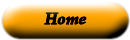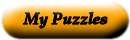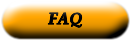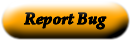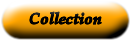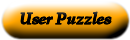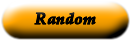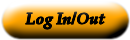Random Sample Rejecting the null hypothesis, Ho, when it is true. Standard error ( ) A sample value used to determine whether to reject the null hypothesis. Sampling distribution sample mean ( ) Range of values constructed from sample data used to estimate a parameter. Point Estimate ( ) A procedure based on sample evidence to determine whether the hypothesis is a reasonable statement. Confidence Intervals ( ) A sample selected so that each item or person in the population has the same chance of being included. Hypothesis ( ) A statement about the value of a population parameter. Hypothesis Testing ( ) A statement about a population parameter developed for the purpose of testing. Null hypothesis ( ) the difference between a sample statistic and its corresponding population parameter. Alternate Hypothesis ( ) A probability distribution of all possible sample means of a given size. Type I Error ( ) A statement that is accepted if the sample data provide sufficient evidence that the null hypothesis is false. Type II Error ( ) The statistic, computed from the sample information, which is used to estimate the population parameter. Test Statistic ( ) Accepting the null hypothesis, Ho, when it is false.

Use the "Printable HTML" button to get a clean page, in either HTML or PDF, that you can use your browser's print button to print. This page won't have buttons or ads, just your puzzle. The PDF format allows the web site to know how large a printer page is, and the fonts are scaled to fill the page. The PDF takes awhile to generate. Don't panic!Web armoredpenguin.com